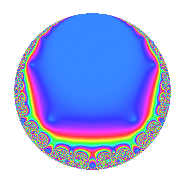# Properties

 Label 9.6.aLevel 9 Weight 6 Character orbit a Rep. character $$\chi_{9}(1,\cdot)$$ Character field $$\Q$$ Dimension 1 Newforms 1 Sturm bound 6 Trace bound 0

# Related objects

## Defining parameters

 Level: $$N$$ = $$9 = 3^{2}$$ Weight: $$k$$ = $$6$$ Character orbit: $$[\chi]$$ = 9.a (trivial) Character field: $$\Q$$ Newforms: $$1$$ Sturm bound: $$6$$ Trace bound: $$0$$

## Dimensions

The following table gives the dimensions of various subspaces of $$M_{6}(\Gamma_0(9))$$.

Total New Old
Modular forms 7 2 5
Cusp forms 3 1 2
Eisenstein series 4 1 3

The following table gives the dimensions of the cuspidal new subspaces with specified eigenvalues for the Atkin-Lehner operators and the Fricke involution.

$$3$$Dim.
$$-$$$$1$$

## Trace form

 $$q$$ $$\mathstrut +\mathstrut 6q^{2}$$ $$\mathstrut +\mathstrut 4q^{4}$$ $$\mathstrut -\mathstrut 6q^{5}$$ $$\mathstrut -\mathstrut 40q^{7}$$ $$\mathstrut -\mathstrut 168q^{8}$$ $$\mathstrut +\mathstrut O(q^{10})$$ $$q$$ $$\mathstrut +\mathstrut 6q^{2}$$ $$\mathstrut +\mathstrut 4q^{4}$$ $$\mathstrut -\mathstrut 6q^{5}$$ $$\mathstrut -\mathstrut 40q^{7}$$ $$\mathstrut -\mathstrut 168q^{8}$$ $$\mathstrut -\mathstrut 36q^{10}$$ $$\mathstrut +\mathstrut 564q^{11}$$ $$\mathstrut +\mathstrut 638q^{13}$$ $$\mathstrut -\mathstrut 240q^{14}$$ $$\mathstrut -\mathstrut 1136q^{16}$$ $$\mathstrut -\mathstrut 882q^{17}$$ $$\mathstrut -\mathstrut 556q^{19}$$ $$\mathstrut -\mathstrut 24q^{20}$$ $$\mathstrut +\mathstrut 3384q^{22}$$ $$\mathstrut +\mathstrut 840q^{23}$$ $$\mathstrut -\mathstrut 3089q^{25}$$ $$\mathstrut +\mathstrut 3828q^{26}$$ $$\mathstrut -\mathstrut 160q^{28}$$ $$\mathstrut -\mathstrut 4638q^{29}$$ $$\mathstrut +\mathstrut 4400q^{31}$$ $$\mathstrut -\mathstrut 1440q^{32}$$ $$\mathstrut -\mathstrut 5292q^{34}$$ $$\mathstrut +\mathstrut 240q^{35}$$ $$\mathstrut -\mathstrut 2410q^{37}$$ $$\mathstrut -\mathstrut 3336q^{38}$$ $$\mathstrut +\mathstrut 1008q^{40}$$ $$\mathstrut +\mathstrut 6870q^{41}$$ $$\mathstrut +\mathstrut 9644q^{43}$$ $$\mathstrut +\mathstrut 2256q^{44}$$ $$\mathstrut +\mathstrut 5040q^{46}$$ $$\mathstrut +\mathstrut 18672q^{47}$$ $$\mathstrut -\mathstrut 15207q^{49}$$ $$\mathstrut -\mathstrut 18534q^{50}$$ $$\mathstrut +\mathstrut 2552q^{52}$$ $$\mathstrut -\mathstrut 33750q^{53}$$ $$\mathstrut -\mathstrut 3384q^{55}$$ $$\mathstrut +\mathstrut 6720q^{56}$$ $$\mathstrut -\mathstrut 27828q^{58}$$ $$\mathstrut +\mathstrut 18084q^{59}$$ $$\mathstrut +\mathstrut 39758q^{61}$$ $$\mathstrut +\mathstrut 26400q^{62}$$ $$\mathstrut +\mathstrut 27712q^{64}$$ $$\mathstrut -\mathstrut 3828q^{65}$$ $$\mathstrut -\mathstrut 23068q^{67}$$ $$\mathstrut -\mathstrut 3528q^{68}$$ $$\mathstrut +\mathstrut 1440q^{70}$$ $$\mathstrut +\mathstrut 4248q^{71}$$ $$\mathstrut -\mathstrut 41110q^{73}$$ $$\mathstrut -\mathstrut 14460q^{74}$$ $$\mathstrut -\mathstrut 2224q^{76}$$ $$\mathstrut -\mathstrut 22560q^{77}$$ $$\mathstrut +\mathstrut 21920q^{79}$$ $$\mathstrut +\mathstrut 6816q^{80}$$ $$\mathstrut +\mathstrut 41220q^{82}$$ $$\mathstrut -\mathstrut 82452q^{83}$$ $$\mathstrut +\mathstrut 5292q^{85}$$ $$\mathstrut +\mathstrut 57864q^{86}$$ $$\mathstrut -\mathstrut 94752q^{88}$$ $$\mathstrut +\mathstrut 94086q^{89}$$ $$\mathstrut -\mathstrut 25520q^{91}$$ $$\mathstrut +\mathstrut 3360q^{92}$$ $$\mathstrut +\mathstrut 112032q^{94}$$ $$\mathstrut +\mathstrut 3336q^{95}$$ $$\mathstrut +\mathstrut 49442q^{97}$$ $$\mathstrut -\mathstrut 91242q^{98}$$ $$\mathstrut +\mathstrut O(q^{100})$$

## Decomposition of $$S_{6}^{\mathrm{new}}(\Gamma_0(9))$$ into irreducible Hecke orbits

Label Dim. $$A$$ Field CM Traces A-L signs $q$-expansion
$$a_2$$ $$a_3$$ $$a_5$$ $$a_7$$ 3
9.6.a.a $$1$$ $$1.443$$ $$\Q$$ None $$6$$ $$0$$ $$-6$$ $$-40$$ $$-$$ $$q+6q^{2}+4q^{4}-6q^{5}-40q^{7}-168q^{8}+\cdots$$

## Decomposition of $$S_{6}^{\mathrm{old}}(\Gamma_0(9))$$ into lower level spaces

$$S_{6}^{\mathrm{old}}(\Gamma_0(9)) \cong$$ $$S_{6}^{\mathrm{new}}(\Gamma_0(3))$$$$^{\oplus 2}$$